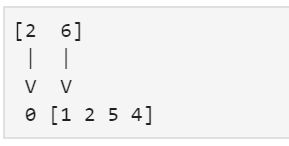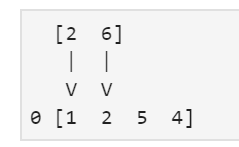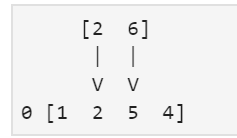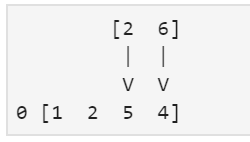Open in App
Not now

# Types of padding in convolution layer

• Difficulty Level : Medium
• Last Updated : 15 Jan, 2019

Let’s discuss padding and its types in convolution layers. In convolution layer we have kernels and to make the final filter more informative we use padding in image matrix or any kind of input array. We have three types of padding that are as follows.

Let’s assume a kernel as a sliding window. We have to come with the solution of padding zeros on the input array. This is a very famous implementation and will be easier to show how it works with a simple example, consider x as a filter and h as an input array.

x[i] = [6, 2]
h[i] = [1, 2, 5, 4]

Using the zero padding, we can calculate the convolution.

You have to invert the filter x, otherwise the operation would be cross-correlation. First step, (now with zero padding):= 2 * 0 + 6 * 1 = 6

Second step:= 2 * 1 + 6 * 2 = 14

Third step:= 2 * 2 + 6 * 5 = 34

Fourth step:= 2 * 5 + 6 * 4 = 34

Fifth step:

= 2 * 4 + 6 * 0 = 8

The result of the convolution for this case, listing all the steps above, would be: Y = [6 14 34 34 8]

 `# importing numpy``import` `numpy as np`` ` `x ``=` `[``6``, ``2``]``h ``=` `[``1``, ``2``, ``5``, ``4``]`` ` `y ``=` `np.convolve(x, h, ``"full"``)``print``(y)  `

Output:

```[ 6 14 34 34  8]
```

In this type of padding, we only append zero to the left of the array and to the top of the 2D input matrix.

 `# importing numpy``import` `numpy as np`` ` `x ``=` `[``6``, ``2``]``h ``=` `[``1``, ``2``, ``5``, ``4``]`` ` `y ``=` `np.convolve(x, h, ``"same"``)``print``(y)`

Output:

```[ 6 14 34 34]
```
 `# importing numpy``import` `numpy as np`` ` `x ``=` `[``6``, ``2``]``h ``=` `[``1``, ``2``, ``5``, ``4``]`` ` `y ``=` `np.convolve(x, h, ``"valid"``)``print``(y)`
```[14 34 34]